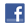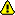New account
4 million accounts created!

• Home
• Report a bug

•

Learn Dutch > Dutch lessons and exercises > Dutch test #22943> Other Dutch exercises on the same topic: Pronunciation [Change theme] > Similar tests: - Pronunciation-sound c/k/s - Spelling - Spelling - Pronunciation-consonants - Pronunciation-diphtongues - Spelling & pronunciation-d / t? - Spelling- ei / ij? - Spelling-g / ch > Double-click on words you don't understand

# Pronunciation

 f + g+ v+ z c = f + f= f + s afgaanafvalof ze k + g+ v+ z c = k + f= k + s ik gaik vindik zeg p + g+ v+ z c = p + f= p + s opgevenop vakantieop zijn s + g+ v+ z c = s + f= s + s t is goedt is veelt is zo t + g+ v+ z c = t + f= t + s voortgaanwat voorzegt ze c + g+ v+ z cc c c weggaanik zeg veelwegzenden

 f + b+ d = v + b= v + d afbetalenof dat k + b+ d = g + b= g + d ik benik doe p + b+ d = b + b= b + d opbellenopdat s + b+ d = z + b= z + d het is beterwie is dat ? t + b+ d = d + b= d + d is dat beter ?ze zegt dat c + b+ d = g + b= g + d ik lig beter zomag dat ?

Examples:

'ik ga', => kx

'zegt ze' => tsTwitter Share
Dutch exercise "Pronunciation" created by mariebru with The test builder. [More lessons & exercises from mariebru]Please log in to save your progress.

1. opvoeren

2. 't is goed

4. wasdoek

5. rustbank

6. het venster

7. zakdoek

8. uitgaan

9. opdagen

10. afzien

End of the free exercise to learn Dutch: Pronunciation
A free Dutch exercise to learn Dutch.
Other Dutch exercises on the same topic : Pronunciation | All our lessons and exercisesShare : Facebook / Twitter / ...